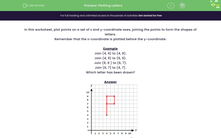# Plotting Letters

In this worksheet, students must plot points on a set of x-y coordinate axes and join certain points to form the shapes of letters.Key stage:  KS 2

Curriculum topic:   Geometry: Position and Direction

Curriculum subtopic:   Plot Points and Draw Sides

Difficulty level:#### Worksheet Overview

In this worksheet, plot points on a set of x and y-coordinate axes, joining the points to form the shapes of letters.

Remember that the x-coordinate is plotted before the y-coordinate.

Example

Join (4, 4) to (4, 9).

Join (4, 9) to (6, 9).

Join (6, 9 ) to (6, 7).

Join (6, 7) to (4, 7).

Which letter has been drawn?